## New Model; New Paradigm Strike fighter. (experimental)

Get some help with creating maps or modding.
Need a map editor or other tools, look here!
Turin86
Greenhorn
Posts: 11
Joined: 25 May 2018, 17:18

###New Model; New Paradigm Strike fighter. (experimental)

This is just a mockup model I made in blender while trying to figure out the workflow of getting a model from blender into Warzone. I've been looking all over for the documentation and can't seem to find enough information...I assume I start with exporting the body and wings seperatly via OBJ files and converting them to PIE format?

The way Im thinking about it I assume the wings would count as their own propulsion system like the VTOL/Helicopter propulsion and the body would be added seperatley, unless they are supposed to be bundled together in the same model file?

Also, After retopology I brought the model down to 2.8k tris... I figure that might be a bit high, still looking for ways to reduce more b/c the final highpoly is about 120k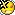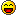thanks for the info in advance.

Berg
RegularPosts: 2202
Joined: 02 Sep 2007, 23:25
Location: Australia

### Re: New Model; New Paradigm Strike fighter. (experimental)

Very pretty you can convert object format to pie with tools in conversion folder or
linux https://sourceforge.net/projects/wzgrap ... s/obj2pie/
win https://sourceforge.net/projects/wzgrap ... j2pie-win/

Turin86
Greenhorn
Posts: 11
Joined: 25 May 2018, 17:18

### Re: New Model; New Paradigm Strike fighter. (experimental)

Berg wrote:Very pretty you can convert object format to pie with tools in conversion folder or
linux https://sourceforge.net/projects/wzgrap ... s/obj2pie/
win https://sourceforge.net/projects/wzgrap ... j2pie-win/

I'm trying to open it through the python console and keep getting the following error...heres the file structure...what am I doing wrong...WZ2100ModsFAn
Trained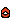Posts: 367
Joined: 15 Apr 2018, 17:25
Location: United States.

### Re: New Model; New Paradigm Strike fighter. (experimental)

Turin86 wrote:
Berg wrote:Very pretty you can convert object format to pie with tools in conversion folder or
linux https://sourceforge.net/projects/wzgrap ... s/obj2pie/
win https://sourceforge.net/projects/wzgrap ... j2pie-win/

I'm trying to open it through the python console and keep getting the following error...heres the file structure...what am I doing wrong...I believe you need to run it manually instead of using something else to run it like Python

try right clicking and left clicking open
I use Ubuntu GamePack By UALinux for gaming. Best Ubuntu Distro for Warzone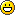Turin86
Greenhorn
Posts: 11
Joined: 25 May 2018, 17:18

### Re: New Model; New Paradigm Strike fighter. (experimental)

WZ2100ModsFAn wrote:
Turin86 wrote:
Berg wrote:Very pretty you can convert object format to pie with tools in conversion folder or
linux https://sourceforge.net/projects/wzgrap ... s/obj2pie/
win https://sourceforge.net/projects/wzgrap ... j2pie-win/

I'm trying to open it through the python console and keep getting the following error...heres the file structure...what am I doing wrong...I believe you need to run it manually instead of using something else to run it like Python

try right clicking and left clicking open

that does not seem to work either... it pops open breifly and closes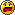WZ2100ModsFAn
TrainedPosts: 367
Joined: 15 Apr 2018, 17:25
Location: United States.

### Re: New Model; New Paradigm Strike fighter. (experimental)

Turin86 wrote:
WZ2100ModsFAn wrote:
Turin86 wrote:
Berg wrote:Very pretty you can convert object format to pie with tools in conversion folder or
linux https://sourceforge.net/projects/wzgrap ... s/obj2pie/
win https://sourceforge.net/projects/wzgrap ... j2pie-win/

I'm trying to open it through the python console and keep getting the following error...heres the file structure...what am I doing wrong...I believe you need to run it manually instead of using something else to run it like Python

try right clicking and left clicking open

that does not seem to work either... it pops open breifly and closesleft click and hold to drag to program.

just drag your objs to this program and open them with it then the conversion will start.

works just like simplipie.

srry brainfarted because i was sleepy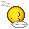I use Ubuntu GamePack By UALinux for gaming. Best Ubuntu Distro for WarzoneBerg
RegularPosts: 2202
Joined: 02 Sep 2007, 23:25
Location: Australia

### Re: New Model; New Paradigm Strike fighter. (experimental)

The original object to pie is in .c follow the instructions to compile and run it which is in this file also.

The python file is a simple user interface and not really needed
// compile command: gcc obj2pie.c -o obj2pie -lm

Code: Select all

``````/*
This file is part of Warzone 2100.
Copyright (C) 2007-2009  Warzone Resurrection Project

Warzone 2100 is free software; you can redistribute it and/or modify
the Free Software Foundation; either version 2 of the License, or
(at your option) any later version.

Warzone 2100 is distributed in the hope that it will be useful,
but WITHOUT ANY WARRANTY; without even the implied warranty of
MERCHANTABILITY or FITNESS FOR A PARTICULAR PURPOSE. See the
GNU General Public License for more details.

You should have received a copy of the GNU General Public License
along with Warzone 2100; if not, write to the Free Software
Foundation, Inc., 51 Franklin St, Fifth Floor, Boston, MA 02110-1301 USA
*/

// compile command: gcc obj2pie.c -o obj2pie -lm

#include <stdio.h>
#include <stdlib.h>
#include <string.h>
#include <math.h>

#ifndef WIN32
#include <stdbool.h>
#include <limits.h>
#else
typedef int bool;
#define PATH_MAX 255
#define true 1
#define false 0
#endif

static char *input_file = "";
static char *output_file = "";
static char *texture_file;
static bool swapYZ = true;
static bool reverseWinding = true;
static bool invertV = true;
static int pieVersion = 3;
static bool twoSided = false;
static unsigned int pieType = 0x200;
static float scaleFactor = 1.0f;

typedef struct _vector3f
{
float x, y, z;
} vector3f;

typedef struct _vector2f
{
float u, v;
} vector2f;

typedef struct _face
{
int index_a_vertex, index_a_texture;
int index_b_vertex, index_b_texture;
int index_c_vertex, index_c_texture;
} face;

static vector3f *points;
static int count_points;

static vector2f *uvcoords;
static int count_uvcoords;

static face *faces;
static int count_faces;

void addpoint(float x, float y, float z)
{
if (points == NULL)
{
points = (vector3f*) malloc(sizeof(vector3f));
count_points = 1;
}
else
{
count_points++;
points = (vector3f*)realloc(points, count_points * sizeof(vector3f));
}

if( pieVersion == 2)
{
points[count_points-1].x = rintf(x);
points[count_points-1].y = rintf(y);
points[count_points-1].z = rintf(z);
}
else // pieVersion == 3
{
points[count_points-1].x = x;
points[count_points-1].y = y;
points[count_points-1].z = z;
}
}

{
if (uvcoords == NULL )
{
uvcoords = (vector2f*) malloc(sizeof(vector2f));
count_uvcoords = 1;
}
else
{
count_uvcoords++;
uvcoords = (vector2f*)realloc(uvcoords, count_uvcoords * sizeof(vector2f));
}

if( pieVersion == 2)
{
uvcoords[count_uvcoords-1].u = rintf(u*256.0f);
uvcoords[count_uvcoords-1].v = rintf(v*256.0f);;
}
else // pieVersion == 3
{
uvcoords[count_uvcoords-1].u = u;
uvcoords[count_uvcoords-1].v = v;
}
}

void addface(int index_a_vertex, int index_a_texture, int index_b_vertex, int index_b_texture, int index_c_vertex, int index_c_texture)
{
if (faces == NULL)
{
faces = (face*) malloc(sizeof(face));
count_faces = 1;
}
else
{
count_faces++;
faces = (face*) realloc(faces, count_faces * sizeof(face));
}
faces[count_faces-1].index_a_vertex = index_a_vertex;
faces[count_faces-1].index_a_texture = index_a_texture;
faces[count_faces-1].index_b_vertex = index_b_vertex;
faces[count_faces-1].index_b_texture = index_b_texture;
faces[count_faces-1].index_c_vertex = index_c_vertex;
faces[count_faces-1].index_c_texture = index_c_texture;
}

{
char buffer;
int index_a_vertex, index_a_texture, index_b_vertex, index_b_texture, index_c_vertex, index_c_texture;
int ignored_a_normal, ignored_b_normal, ignored_c_normal;
float u, v;
float x, y, z;
bool normalsDetected = false;

if (input == NULL)
{
return;
}

points = NULL;
count_points = 0;

uvcoords = NULL;
count_uvcoords = 0;

faces = NULL;
count_faces = 0;

while (fgets(buffer, 256, input) != NULL)
{
if (buffer == 'v' && buffer == 'n')
{
normalsDetected = true;
}
else if (buffer == 'v' && buffer == 't')
{
sscanf(&buffer, "%f %f", &u, &v);
if (invertV)
v = 1.0f - v;
}
else if (buffer == 'v')		// && buffer != 't' && buffer != 'n'
{
sscanf(&buffer, "%f %f %f", &x, &y, &z);
(swapYZ?z:y)*scaleFactor,
(swapYZ?y:z)*scaleFactor);
}
else if (buffer == 'f' )
{
if(normalsDetected)
{
sscanf(&buffer, "%d/%d/%d %d/%d/%d %d/%d/%d",
&index_a_vertex, &index_a_texture, &ignored_a_normal,
&index_b_vertex, &index_b_texture, &ignored_b_normal,
&index_c_vertex, &index_c_texture, &ignored_c_normal);
}
else
{
sscanf(&buffer, "%d/%d %d/%d %d/%d",
&index_a_vertex, &index_a_texture,
&index_b_vertex, &index_b_texture,
&index_c_vertex, &index_c_texture);
}

if(reverseWinding || twoSided)
{
addface(index_c_vertex - 1, index_c_texture - 1,
index_b_vertex - 1, index_b_texture - 1,
index_a_vertex - 1, index_a_texture - 1);
}
if(!reverseWinding || twoSided)
{
addface(index_a_vertex - 1, index_a_texture - 1,
index_b_vertex - 1, index_b_texture - 1,
index_c_vertex - 1, index_c_texture - 1);
}
}
}
}

void writepie(FILE *output)
{
size_t i;

if (output == NULL)
{
return;
}

fprintf(output, "PIE %d\nTYPE %x\n", pieVersion, pieType);
fprintf(output, "TEXTURE 0 %s 256 256\n", texture_file);
fprintf(output, "LEVELS 1\nLEVEL 1\n");

fprintf(output, "POINTS %d\n", count_points);
for (i = 0;i < count_points;i++)
{
if( pieVersion == 2)
{
fprintf(output, "\t%d %d %d\n", (int)points[i].x, (int)points[i].y, (int)points[i].z);
}
else // pieVersion == 3
{
fprintf(output, "\t%f %f %f\n", points[i].x, points[i].y, points[i].z);
}
}

fprintf(output, "POLYGONS %d\n", count_faces);
for (i = 0;i < count_faces;i++)
{
if(pieVersion == 2)
{
fprintf(output, "\t200 3 %d %d %d %d %d %d %d %d %d\n",
faces[i].index_a_vertex,
faces[i].index_b_vertex,
faces[i].index_c_vertex,
(int)uvcoords[faces[i].index_a_texture].u,
(int)uvcoords[faces[i].index_a_texture].v,
(int)uvcoords[faces[i].index_b_texture].u,
(int)uvcoords[faces[i].index_b_texture].v,
(int)uvcoords[faces[i].index_c_texture].u,
(int)uvcoords[faces[i].index_c_texture].v);
}
else // pieVersion == 3
{
fprintf(output, "\t200 3 %d %d %d %f %f %f %f %f %f\n",
faces[i].index_a_vertex,
faces[i].index_b_vertex,
faces[i].index_c_vertex,
uvcoords[faces[i].index_a_texture].u,
uvcoords[faces[i].index_a_texture].v,
uvcoords[faces[i].index_b_texture].u,
uvcoords[faces[i].index_b_texture].v,
uvcoords[faces[i].index_c_texture].u,
uvcoords[faces[i].index_c_texture].v);
}
}
}

void objtopie()
{
FILE *input = NULL;
FILE *output = NULL;

input = fopen(input_file, "rt");
if (input == NULL)
{
perror("Couldn't open input file");
return;
}

output = fopen(output_file, "wt");
if (output == NULL)
{
perror("Couldn't open output file");
fclose(input);
return;
}

writepie(output);

fclose(input);
fclose(output);
}

static void parse_args(int argc, char **argv)
{
unsigned int i = 1;

for (i = 1; argc >= 3 + i && argv[i] == '-'; i++)
{
if (argv[i] == 'y')
{
swapYZ = false; // exporting program used Y-axis as "up", like we do, don't switch
}
else if (argv[i] == 'i')
{
invertV = false;
}
else if (argv[i] == 't')
{
twoSided = true;
}
else if (argv[i] == 'r')
{
reverseWinding = false;
}
else if (argv[i] == 's')
{
int ret;

i++;
if (argc < i)
{
fprintf(stderr, "Missing parameter to scale option.\n");
exit(1);
}
ret = sscanf(argv[i], "%f", &scaleFactor);
if (ret != 1)
{
fprintf(stderr, "Bad parameter to scale option.\n");
exit(1);
}
}
else if (argv[i] == 'v')
{
int ret;

i++;
if (argc < i)
{
fprintf(stderr, "Missing parameter to pie version option.\n");
exit(1);
}
ret = sscanf(argv[i], "%d", &pieVersion);
if (ret != 1)
{
fprintf(stderr, "Bad parameter to pie version option.\n");
exit(1);
}
if( pieVersion < 2 || pieVersion > 3)
{
fprintf(stderr, "Unsupported pie version %d.\n",pieVersion);
exit(1);
}
}
else if (argv[i] == 'm')
{
pieType |= 0x10000;
}
else
{
fprintf(stderr, "Unrecognized option: %s\n", argv[i]);
exit(1);
}
}
if (argc < 3 + i)
{
fprintf(stderr, "Usage: obj2pie [options] input_filename output_filename texture_filename\n");
fprintf(stderr, "Options:\n");
fprintf(stderr, "  -y		Do not swap Y and Z axis. Exporter uses Y-axis as \"up\".\n");
fprintf(stderr, "  -r		Do not reverse winding of all polygons.\n");
fprintf(stderr, "  -i		Do not invert the vertical texture coordinates.\n");
fprintf(stderr, "  -s N		Scale model points by N before converting.\n");
fprintf(stderr, "  -t		Use two sided polygons (Create duplicate faces with reverse winding).\n");
fprintf(stderr, "  -v N		Pie export version ( 2 and 3 supported, 3 is default).\n");
fprintf(stderr, "  -m		Use tcmask pie type.\n");

exit(1);
}
input_file = argv[i++];
output_file = argv[i++];
texture_file = argv[i++];
}

int main(int argc, char *argv[])
{
parse_args(argc, argv);
objtopie();
return 0;
}``````

WZ2100ModsFAn
TrainedPosts: 367
Joined: 15 Apr 2018, 17:25
Location: United States.

### Re: New Model; New Paradigm Strike fighter. (experimental)

Berg wrote:The original object to pie is in .c follow the instructions to compile and run it which is in this file also.

The python file is a simple user interface and not really needed
// compile command: gcc obj2pie.c -o obj2pie -lm

Code: Select all

``````/*
This file is part of Warzone 2100.
Copyright (C) 2007-2009  Warzone Resurrection Project

Warzone 2100 is free software; you can redistribute it and/or modify
the Free Software Foundation; either version 2 of the License, or
(at your option) any later version.

Warzone 2100 is distributed in the hope that it will be useful,
but WITHOUT ANY WARRANTY; without even the implied warranty of
MERCHANTABILITY or FITNESS FOR A PARTICULAR PURPOSE. See the
GNU General Public License for more details.

You should have received a copy of the GNU General Public License
along with Warzone 2100; if not, write to the Free Software
Foundation, Inc., 51 Franklin St, Fifth Floor, Boston, MA 02110-1301 USA
*/

// compile command: gcc obj2pie.c -o obj2pie -lm

#include <stdio.h>
#include <stdlib.h>
#include <string.h>
#include <math.h>

#ifndef WIN32
#include <stdbool.h>
#include <limits.h>
#else
typedef int bool;
#define PATH_MAX 255
#define true 1
#define false 0
#endif

static char *input_file = "";
static char *output_file = "";
static char *texture_file;
static bool swapYZ = true;
static bool reverseWinding = true;
static bool invertV = true;
static int pieVersion = 3;
static bool twoSided = false;
static unsigned int pieType = 0x200;
static float scaleFactor = 1.0f;

typedef struct _vector3f
{
float x, y, z;
} vector3f;

typedef struct _vector2f
{
float u, v;
} vector2f;

typedef struct _face
{
int index_a_vertex, index_a_texture;
int index_b_vertex, index_b_texture;
int index_c_vertex, index_c_texture;
} face;

static vector3f *points;
static int count_points;

static vector2f *uvcoords;
static int count_uvcoords;

static face *faces;
static int count_faces;

void addpoint(float x, float y, float z)
{
if (points == NULL)
{
points = (vector3f*) malloc(sizeof(vector3f));
count_points = 1;
}
else
{
count_points++;
points = (vector3f*)realloc(points, count_points * sizeof(vector3f));
}

if( pieVersion == 2)
{
points[count_points-1].x = rintf(x);
points[count_points-1].y = rintf(y);
points[count_points-1].z = rintf(z);
}
else // pieVersion == 3
{
points[count_points-1].x = x;
points[count_points-1].y = y;
points[count_points-1].z = z;
}
}

{
if (uvcoords == NULL )
{
uvcoords = (vector2f*) malloc(sizeof(vector2f));
count_uvcoords = 1;
}
else
{
count_uvcoords++;
uvcoords = (vector2f*)realloc(uvcoords, count_uvcoords * sizeof(vector2f));
}

if( pieVersion == 2)
{
uvcoords[count_uvcoords-1].u = rintf(u*256.0f);
uvcoords[count_uvcoords-1].v = rintf(v*256.0f);;
}
else // pieVersion == 3
{
uvcoords[count_uvcoords-1].u = u;
uvcoords[count_uvcoords-1].v = v;
}
}

void addface(int index_a_vertex, int index_a_texture, int index_b_vertex, int index_b_texture, int index_c_vertex, int index_c_texture)
{
if (faces == NULL)
{
faces = (face*) malloc(sizeof(face));
count_faces = 1;
}
else
{
count_faces++;
faces = (face*) realloc(faces, count_faces * sizeof(face));
}
faces[count_faces-1].index_a_vertex = index_a_vertex;
faces[count_faces-1].index_a_texture = index_a_texture;
faces[count_faces-1].index_b_vertex = index_b_vertex;
faces[count_faces-1].index_b_texture = index_b_texture;
faces[count_faces-1].index_c_vertex = index_c_vertex;
faces[count_faces-1].index_c_texture = index_c_texture;
}

{
char buffer;
int index_a_vertex, index_a_texture, index_b_vertex, index_b_texture, index_c_vertex, index_c_texture;
int ignored_a_normal, ignored_b_normal, ignored_c_normal;
float u, v;
float x, y, z;
bool normalsDetected = false;

if (input == NULL)
{
return;
}

points = NULL;
count_points = 0;

uvcoords = NULL;
count_uvcoords = 0;

faces = NULL;
count_faces = 0;

while (fgets(buffer, 256, input) != NULL)
{
if (buffer == 'v' && buffer == 'n')
{
normalsDetected = true;
}
else if (buffer == 'v' && buffer == 't')
{
sscanf(&buffer, "%f %f", &u, &v);
if (invertV)
v = 1.0f - v;
}
else if (buffer == 'v')		// && buffer != 't' && buffer != 'n'
{
sscanf(&buffer, "%f %f %f", &x, &y, &z);
(swapYZ?z:y)*scaleFactor,
(swapYZ?y:z)*scaleFactor);
}
else if (buffer == 'f' )
{
if(normalsDetected)
{
sscanf(&buffer, "%d/%d/%d %d/%d/%d %d/%d/%d",
&index_a_vertex, &index_a_texture, &ignored_a_normal,
&index_b_vertex, &index_b_texture, &ignored_b_normal,
&index_c_vertex, &index_c_texture, &ignored_c_normal);
}
else
{
sscanf(&buffer, "%d/%d %d/%d %d/%d",
&index_a_vertex, &index_a_texture,
&index_b_vertex, &index_b_texture,
&index_c_vertex, &index_c_texture);
}

if(reverseWinding || twoSided)
{
addface(index_c_vertex - 1, index_c_texture - 1,
index_b_vertex - 1, index_b_texture - 1,
index_a_vertex - 1, index_a_texture - 1);
}
if(!reverseWinding || twoSided)
{
addface(index_a_vertex - 1, index_a_texture - 1,
index_b_vertex - 1, index_b_texture - 1,
index_c_vertex - 1, index_c_texture - 1);
}
}
}
}

void writepie(FILE *output)
{
size_t i;

if (output == NULL)
{
return;
}

fprintf(output, "PIE %d\nTYPE %x\n", pieVersion, pieType);
fprintf(output, "TEXTURE 0 %s 256 256\n", texture_file);
fprintf(output, "LEVELS 1\nLEVEL 1\n");

fprintf(output, "POINTS %d\n", count_points);
for (i = 0;i < count_points;i++)
{
if( pieVersion == 2)
{
fprintf(output, "\t%d %d %d\n", (int)points[i].x, (int)points[i].y, (int)points[i].z);
}
else // pieVersion == 3
{
fprintf(output, "\t%f %f %f\n", points[i].x, points[i].y, points[i].z);
}
}

fprintf(output, "POLYGONS %d\n", count_faces);
for (i = 0;i < count_faces;i++)
{
if(pieVersion == 2)
{
fprintf(output, "\t200 3 %d %d %d %d %d %d %d %d %d\n",
faces[i].index_a_vertex,
faces[i].index_b_vertex,
faces[i].index_c_vertex,
(int)uvcoords[faces[i].index_a_texture].u,
(int)uvcoords[faces[i].index_a_texture].v,
(int)uvcoords[faces[i].index_b_texture].u,
(int)uvcoords[faces[i].index_b_texture].v,
(int)uvcoords[faces[i].index_c_texture].u,
(int)uvcoords[faces[i].index_c_texture].v);
}
else // pieVersion == 3
{
fprintf(output, "\t200 3 %d %d %d %f %f %f %f %f %f\n",
faces[i].index_a_vertex,
faces[i].index_b_vertex,
faces[i].index_c_vertex,
uvcoords[faces[i].index_a_texture].u,
uvcoords[faces[i].index_a_texture].v,
uvcoords[faces[i].index_b_texture].u,
uvcoords[faces[i].index_b_texture].v,
uvcoords[faces[i].index_c_texture].u,
uvcoords[faces[i].index_c_texture].v);
}
}
}

void objtopie()
{
FILE *input = NULL;
FILE *output = NULL;

input = fopen(input_file, "rt");
if (input == NULL)
{
perror("Couldn't open input file");
return;
}

output = fopen(output_file, "wt");
if (output == NULL)
{
perror("Couldn't open output file");
fclose(input);
return;
}

writepie(output);

fclose(input);
fclose(output);
}

static void parse_args(int argc, char **argv)
{
unsigned int i = 1;

for (i = 1; argc >= 3 + i && argv[i] == '-'; i++)
{
if (argv[i] == 'y')
{
swapYZ = false; // exporting program used Y-axis as "up", like we do, don't switch
}
else if (argv[i] == 'i')
{
invertV = false;
}
else if (argv[i] == 't')
{
twoSided = true;
}
else if (argv[i] == 'r')
{
reverseWinding = false;
}
else if (argv[i] == 's')
{
int ret;

i++;
if (argc < i)
{
fprintf(stderr, "Missing parameter to scale option.\n");
exit(1);
}
ret = sscanf(argv[i], "%f", &scaleFactor);
if (ret != 1)
{
fprintf(stderr, "Bad parameter to scale option.\n");
exit(1);
}
}
else if (argv[i] == 'v')
{
int ret;

i++;
if (argc < i)
{
fprintf(stderr, "Missing parameter to pie version option.\n");
exit(1);
}
ret = sscanf(argv[i], "%d", &pieVersion);
if (ret != 1)
{
fprintf(stderr, "Bad parameter to pie version option.\n");
exit(1);
}
if( pieVersion < 2 || pieVersion > 3)
{
fprintf(stderr, "Unsupported pie version %d.\n",pieVersion);
exit(1);
}
}
else if (argv[i] == 'm')
{
pieType |= 0x10000;
}
else
{
fprintf(stderr, "Unrecognized option: %s\n", argv[i]);
exit(1);
}
}
if (argc < 3 + i)
{
fprintf(stderr, "Usage: obj2pie [options] input_filename output_filename texture_filename\n");
fprintf(stderr, "Options:\n");
fprintf(stderr, "  -y		Do not swap Y and Z axis. Exporter uses Y-axis as \"up\".\n");
fprintf(stderr, "  -r		Do not reverse winding of all polygons.\n");
fprintf(stderr, "  -i		Do not invert the vertical texture coordinates.\n");
fprintf(stderr, "  -s N		Scale model points by N before converting.\n");
fprintf(stderr, "  -t		Use two sided polygons (Create duplicate faces with reverse winding).\n");
fprintf(stderr, "  -v N		Pie export version ( 2 and 3 supported, 3 is default).\n");
fprintf(stderr, "  -m		Use tcmask pie type.\n");

exit(1);
}
input_file = argv[i++];
output_file = argv[i++];
texture_file = argv[i++];
}

int main(int argc, char *argv[])
{
parse_args(argc, argv);
objtopie();
return 0;
}``````
I'll switch to debian on that case thank you berg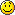I use Ubuntu GamePack By UALinux for gaming. Best Ubuntu Distro for WarzoneTurin86
Greenhorn
Posts: 11
Joined: 25 May 2018, 17:18

### Re: New Model; New Paradigm Strike fighter. (experimental)

Berg wrote:The original object to pie is in .c follow the instructions to compile and run it which is in this file also.

The python file is a simple user interface and not really needed
// compile command: gcc obj2pie.c -o obj2pie -lm

Code: Select all

``````/*
This file is part of Warzone 2100.
Copyright (C) 2007-2009  Warzone Resurrection Project

Warzone 2100 is free software; you can redistribute it and/or modify
the Free Software Foundation; either version 2 of the License, or
(at your option) any later version.

Warzone 2100 is distributed in the hope that it will be useful,
but WITHOUT ANY WARRANTY; without even the implied warranty of
MERCHANTABILITY or FITNESS FOR A PARTICULAR PURPOSE. See the
GNU General Public License for more details.

You should have received a copy of the GNU General Public License
along with Warzone 2100; if not, write to the Free Software
Foundation, Inc., 51 Franklin St, Fifth Floor, Boston, MA 02110-1301 USA
*/

// compile command: gcc obj2pie.c -o obj2pie -lm

#include <stdio.h>
#include <stdlib.h>
#include <string.h>
#include <math.h>

#ifndef WIN32
#include <stdbool.h>
#include <limits.h>
#else
typedef int bool;
#define PATH_MAX 255
#define true 1
#define false 0
#endif

static char *input_file = "";
static char *output_file = "";
static char *texture_file;
static bool swapYZ = true;
static bool reverseWinding = true;
static bool invertV = true;
static int pieVersion = 3;
static bool twoSided = false;
static unsigned int pieType = 0x200;
static float scaleFactor = 1.0f;

typedef struct _vector3f
{
float x, y, z;
} vector3f;

typedef struct _vector2f
{
float u, v;
} vector2f;

typedef struct _face
{
int index_a_vertex, index_a_texture;
int index_b_vertex, index_b_texture;
int index_c_vertex, index_c_texture;
} face;

static vector3f *points;
static int count_points;

static vector2f *uvcoords;
static int count_uvcoords;

static face *faces;
static int count_faces;

void addpoint(float x, float y, float z)
{
if (points == NULL)
{
points = (vector3f*) malloc(sizeof(vector3f));
count_points = 1;
}
else
{
count_points++;
points = (vector3f*)realloc(points, count_points * sizeof(vector3f));
}

if( pieVersion == 2)
{
points[count_points-1].x = rintf(x);
points[count_points-1].y = rintf(y);
points[count_points-1].z = rintf(z);
}
else // pieVersion == 3
{
points[count_points-1].x = x;
points[count_points-1].y = y;
points[count_points-1].z = z;
}
}

{
if (uvcoords == NULL )
{
uvcoords = (vector2f*) malloc(sizeof(vector2f));
count_uvcoords = 1;
}
else
{
count_uvcoords++;
uvcoords = (vector2f*)realloc(uvcoords, count_uvcoords * sizeof(vector2f));
}

if( pieVersion == 2)
{
uvcoords[count_uvcoords-1].u = rintf(u*256.0f);
uvcoords[count_uvcoords-1].v = rintf(v*256.0f);;
}
else // pieVersion == 3
{
uvcoords[count_uvcoords-1].u = u;
uvcoords[count_uvcoords-1].v = v;
}
}

void addface(int index_a_vertex, int index_a_texture, int index_b_vertex, int index_b_texture, int index_c_vertex, int index_c_texture)
{
if (faces == NULL)
{
faces = (face*) malloc(sizeof(face));
count_faces = 1;
}
else
{
count_faces++;
faces = (face*) realloc(faces, count_faces * sizeof(face));
}
faces[count_faces-1].index_a_vertex = index_a_vertex;
faces[count_faces-1].index_a_texture = index_a_texture;
faces[count_faces-1].index_b_vertex = index_b_vertex;
faces[count_faces-1].index_b_texture = index_b_texture;
faces[count_faces-1].index_c_vertex = index_c_vertex;
faces[count_faces-1].index_c_texture = index_c_texture;
}

{
char buffer;
int index_a_vertex, index_a_texture, index_b_vertex, index_b_texture, index_c_vertex, index_c_texture;
int ignored_a_normal, ignored_b_normal, ignored_c_normal;
float u, v;
float x, y, z;
bool normalsDetected = false;

if (input == NULL)
{
return;
}

points = NULL;
count_points = 0;

uvcoords = NULL;
count_uvcoords = 0;

faces = NULL;
count_faces = 0;

while (fgets(buffer, 256, input) != NULL)
{
if (buffer == 'v' && buffer == 'n')
{
normalsDetected = true;
}
else if (buffer == 'v' && buffer == 't')
{
sscanf(&buffer, "%f %f", &u, &v);
if (invertV)
v = 1.0f - v;
}
else if (buffer == 'v')		// && buffer != 't' && buffer != 'n'
{
sscanf(&buffer, "%f %f %f", &x, &y, &z);
(swapYZ?z:y)*scaleFactor,
(swapYZ?y:z)*scaleFactor);
}
else if (buffer == 'f' )
{
if(normalsDetected)
{
sscanf(&buffer, "%d/%d/%d %d/%d/%d %d/%d/%d",
&index_a_vertex, &index_a_texture, &ignored_a_normal,
&index_b_vertex, &index_b_texture, &ignored_b_normal,
&index_c_vertex, &index_c_texture, &ignored_c_normal);
}
else
{
sscanf(&buffer, "%d/%d %d/%d %d/%d",
&index_a_vertex, &index_a_texture,
&index_b_vertex, &index_b_texture,
&index_c_vertex, &index_c_texture);
}

if(reverseWinding || twoSided)
{
addface(index_c_vertex - 1, index_c_texture - 1,
index_b_vertex - 1, index_b_texture - 1,
index_a_vertex - 1, index_a_texture - 1);
}
if(!reverseWinding || twoSided)
{
addface(index_a_vertex - 1, index_a_texture - 1,
index_b_vertex - 1, index_b_texture - 1,
index_c_vertex - 1, index_c_texture - 1);
}
}
}
}

void writepie(FILE *output)
{
size_t i;

if (output == NULL)
{
return;
}

fprintf(output, "PIE %d\nTYPE %x\n", pieVersion, pieType);
fprintf(output, "TEXTURE 0 %s 256 256\n", texture_file);
fprintf(output, "LEVELS 1\nLEVEL 1\n");

fprintf(output, "POINTS %d\n", count_points);
for (i = 0;i < count_points;i++)
{
if( pieVersion == 2)
{
fprintf(output, "\t%d %d %d\n", (int)points[i].x, (int)points[i].y, (int)points[i].z);
}
else // pieVersion == 3
{
fprintf(output, "\t%f %f %f\n", points[i].x, points[i].y, points[i].z);
}
}

fprintf(output, "POLYGONS %d\n", count_faces);
for (i = 0;i < count_faces;i++)
{
if(pieVersion == 2)
{
fprintf(output, "\t200 3 %d %d %d %d %d %d %d %d %d\n",
faces[i].index_a_vertex,
faces[i].index_b_vertex,
faces[i].index_c_vertex,
(int)uvcoords[faces[i].index_a_texture].u,
(int)uvcoords[faces[i].index_a_texture].v,
(int)uvcoords[faces[i].index_b_texture].u,
(int)uvcoords[faces[i].index_b_texture].v,
(int)uvcoords[faces[i].index_c_texture].u,
(int)uvcoords[faces[i].index_c_texture].v);
}
else // pieVersion == 3
{
fprintf(output, "\t200 3 %d %d %d %f %f %f %f %f %f\n",
faces[i].index_a_vertex,
faces[i].index_b_vertex,
faces[i].index_c_vertex,
uvcoords[faces[i].index_a_texture].u,
uvcoords[faces[i].index_a_texture].v,
uvcoords[faces[i].index_b_texture].u,
uvcoords[faces[i].index_b_texture].v,
uvcoords[faces[i].index_c_texture].u,
uvcoords[faces[i].index_c_texture].v);
}
}
}

void objtopie()
{
FILE *input = NULL;
FILE *output = NULL;

input = fopen(input_file, "rt");
if (input == NULL)
{
perror("Couldn't open input file");
return;
}

output = fopen(output_file, "wt");
if (output == NULL)
{
perror("Couldn't open output file");
fclose(input);
return;
}

writepie(output);

fclose(input);
fclose(output);
}

static void parse_args(int argc, char **argv)
{
unsigned int i = 1;

for (i = 1; argc >= 3 + i && argv[i] == '-'; i++)
{
if (argv[i] == 'y')
{
swapYZ = false; // exporting program used Y-axis as "up", like we do, don't switch
}
else if (argv[i] == 'i')
{
invertV = false;
}
else if (argv[i] == 't')
{
twoSided = true;
}
else if (argv[i] == 'r')
{
reverseWinding = false;
}
else if (argv[i] == 's')
{
int ret;

i++;
if (argc < i)
{
fprintf(stderr, "Missing parameter to scale option.\n");
exit(1);
}
ret = sscanf(argv[i], "%f", &scaleFactor);
if (ret != 1)
{
fprintf(stderr, "Bad parameter to scale option.\n");
exit(1);
}
}
else if (argv[i] == 'v')
{
int ret;

i++;
if (argc < i)
{
fprintf(stderr, "Missing parameter to pie version option.\n");
exit(1);
}
ret = sscanf(argv[i], "%d", &pieVersion);
if (ret != 1)
{
fprintf(stderr, "Bad parameter to pie version option.\n");
exit(1);
}
if( pieVersion < 2 || pieVersion > 3)
{
fprintf(stderr, "Unsupported pie version %d.\n",pieVersion);
exit(1);
}
}
else if (argv[i] == 'm')
{
pieType |= 0x10000;
}
else
{
fprintf(stderr, "Unrecognized option: %s\n", argv[i]);
exit(1);
}
}
if (argc < 3 + i)
{
fprintf(stderr, "Usage: obj2pie [options] input_filename output_filename texture_filename\n");
fprintf(stderr, "Options:\n");
fprintf(stderr, "  -y		Do not swap Y and Z axis. Exporter uses Y-axis as \"up\".\n");
fprintf(stderr, "  -r		Do not reverse winding of all polygons.\n");
fprintf(stderr, "  -i		Do not invert the vertical texture coordinates.\n");
fprintf(stderr, "  -s N		Scale model points by N before converting.\n");
fprintf(stderr, "  -t		Use two sided polygons (Create duplicate faces with reverse winding).\n");
fprintf(stderr, "  -v N		Pie export version ( 2 and 3 supported, 3 is default).\n");
fprintf(stderr, "  -m		Use tcmask pie type.\n");

exit(1);
}
input_file = argv[i++];
output_file = argv[i++];
texture_file = argv[i++];
}

int main(int argc, char *argv[])
{
parse_args(argc, argv);
objtopie();
return 0;
}``````

I did the command and was not able to open the interface...WZ2100ModsFAn
TrainedPosts: 367
Joined: 15 Apr 2018, 17:25
Location: United States.

### Re: New Model; New Paradigm Strike fighter. (experimental)

Turin86 wrote:
Berg wrote:The original object to pie is in .c follow the instructions to compile and run it which is in this file also.

The python file is a simple user interface and not really needed
// compile command: gcc obj2pie.c -o obj2pie -lm

Code: Select all

``````/*
This file is part of Warzone 2100.
Copyright (C) 2007-2009  Warzone Resurrection Project

Warzone 2100 is free software; you can redistribute it and/or modify
the Free Software Foundation; either version 2 of the License, or
(at your option) any later version.

Warzone 2100 is distributed in the hope that it will be useful,
but WITHOUT ANY WARRANTY; without even the implied warranty of
MERCHANTABILITY or FITNESS FOR A PARTICULAR PURPOSE. See the
GNU General Public License for more details.

You should have received a copy of the GNU General Public License
along with Warzone 2100; if not, write to the Free Software
Foundation, Inc., 51 Franklin St, Fifth Floor, Boston, MA 02110-1301 USA
*/

// compile command: gcc obj2pie.c -o obj2pie -lm

#include <stdio.h>
#include <stdlib.h>
#include <string.h>
#include <math.h>

#ifndef WIN32
#include <stdbool.h>
#include <limits.h>
#else
typedef int bool;
#define PATH_MAX 255
#define true 1
#define false 0
#endif

static char *input_file = "";
static char *output_file = "";
static char *texture_file;
static bool swapYZ = true;
static bool reverseWinding = true;
static bool invertV = true;
static int pieVersion = 3;
static bool twoSided = false;
static unsigned int pieType = 0x200;
static float scaleFactor = 1.0f;

typedef struct _vector3f
{
float x, y, z;
} vector3f;

typedef struct _vector2f
{
float u, v;
} vector2f;

typedef struct _face
{
int index_a_vertex, index_a_texture;
int index_b_vertex, index_b_texture;
int index_c_vertex, index_c_texture;
} face;

static vector3f *points;
static int count_points;

static vector2f *uvcoords;
static int count_uvcoords;

static face *faces;
static int count_faces;

void addpoint(float x, float y, float z)
{
if (points == NULL)
{
points = (vector3f*) malloc(sizeof(vector3f));
count_points = 1;
}
else
{
count_points++;
points = (vector3f*)realloc(points, count_points * sizeof(vector3f));
}

if( pieVersion == 2)
{
points[count_points-1].x = rintf(x);
points[count_points-1].y = rintf(y);
points[count_points-1].z = rintf(z);
}
else // pieVersion == 3
{
points[count_points-1].x = x;
points[count_points-1].y = y;
points[count_points-1].z = z;
}
}

{
if (uvcoords == NULL )
{
uvcoords = (vector2f*) malloc(sizeof(vector2f));
count_uvcoords = 1;
}
else
{
count_uvcoords++;
uvcoords = (vector2f*)realloc(uvcoords, count_uvcoords * sizeof(vector2f));
}

if( pieVersion == 2)
{
uvcoords[count_uvcoords-1].u = rintf(u*256.0f);
uvcoords[count_uvcoords-1].v = rintf(v*256.0f);;
}
else // pieVersion == 3
{
uvcoords[count_uvcoords-1].u = u;
uvcoords[count_uvcoords-1].v = v;
}
}

void addface(int index_a_vertex, int index_a_texture, int index_b_vertex, int index_b_texture, int index_c_vertex, int index_c_texture)
{
if (faces == NULL)
{
faces = (face*) malloc(sizeof(face));
count_faces = 1;
}
else
{
count_faces++;
faces = (face*) realloc(faces, count_faces * sizeof(face));
}
faces[count_faces-1].index_a_vertex = index_a_vertex;
faces[count_faces-1].index_a_texture = index_a_texture;
faces[count_faces-1].index_b_vertex = index_b_vertex;
faces[count_faces-1].index_b_texture = index_b_texture;
faces[count_faces-1].index_c_vertex = index_c_vertex;
faces[count_faces-1].index_c_texture = index_c_texture;
}

{
char buffer;
int index_a_vertex, index_a_texture, index_b_vertex, index_b_texture, index_c_vertex, index_c_texture;
int ignored_a_normal, ignored_b_normal, ignored_c_normal;
float u, v;
float x, y, z;
bool normalsDetected = false;

if (input == NULL)
{
return;
}

points = NULL;
count_points = 0;

uvcoords = NULL;
count_uvcoords = 0;

faces = NULL;
count_faces = 0;

while (fgets(buffer, 256, input) != NULL)
{
if (buffer == 'v' && buffer == 'n')
{
normalsDetected = true;
}
else if (buffer == 'v' && buffer == 't')
{
sscanf(&buffer, "%f %f", &u, &v);
if (invertV)
v = 1.0f - v;
}
else if (buffer == 'v')		// && buffer != 't' && buffer != 'n'
{
sscanf(&buffer, "%f %f %f", &x, &y, &z);
(swapYZ?z:y)*scaleFactor,
(swapYZ?y:z)*scaleFactor);
}
else if (buffer == 'f' )
{
if(normalsDetected)
{
sscanf(&buffer, "%d/%d/%d %d/%d/%d %d/%d/%d",
&index_a_vertex, &index_a_texture, &ignored_a_normal,
&index_b_vertex, &index_b_texture, &ignored_b_normal,
&index_c_vertex, &index_c_texture, &ignored_c_normal);
}
else
{
sscanf(&buffer, "%d/%d %d/%d %d/%d",
&index_a_vertex, &index_a_texture,
&index_b_vertex, &index_b_texture,
&index_c_vertex, &index_c_texture);
}

if(reverseWinding || twoSided)
{
addface(index_c_vertex - 1, index_c_texture - 1,
index_b_vertex - 1, index_b_texture - 1,
index_a_vertex - 1, index_a_texture - 1);
}
if(!reverseWinding || twoSided)
{
addface(index_a_vertex - 1, index_a_texture - 1,
index_b_vertex - 1, index_b_texture - 1,
index_c_vertex - 1, index_c_texture - 1);
}
}
}
}

void writepie(FILE *output)
{
size_t i;

if (output == NULL)
{
return;
}

fprintf(output, "PIE %d\nTYPE %x\n", pieVersion, pieType);
fprintf(output, "TEXTURE 0 %s 256 256\n", texture_file);
fprintf(output, "LEVELS 1\nLEVEL 1\n");

fprintf(output, "POINTS %d\n", count_points);
for (i = 0;i < count_points;i++)
{
if( pieVersion == 2)
{
fprintf(output, "\t%d %d %d\n", (int)points[i].x, (int)points[i].y, (int)points[i].z);
}
else // pieVersion == 3
{
fprintf(output, "\t%f %f %f\n", points[i].x, points[i].y, points[i].z);
}
}

fprintf(output, "POLYGONS %d\n", count_faces);
for (i = 0;i < count_faces;i++)
{
if(pieVersion == 2)
{
fprintf(output, "\t200 3 %d %d %d %d %d %d %d %d %d\n",
faces[i].index_a_vertex,
faces[i].index_b_vertex,
faces[i].index_c_vertex,
(int)uvcoords[faces[i].index_a_texture].u,
(int)uvcoords[faces[i].index_a_texture].v,
(int)uvcoords[faces[i].index_b_texture].u,
(int)uvcoords[faces[i].index_b_texture].v,
(int)uvcoords[faces[i].index_c_texture].u,
(int)uvcoords[faces[i].index_c_texture].v);
}
else // pieVersion == 3
{
fprintf(output, "\t200 3 %d %d %d %f %f %f %f %f %f\n",
faces[i].index_a_vertex,
faces[i].index_b_vertex,
faces[i].index_c_vertex,
uvcoords[faces[i].index_a_texture].u,
uvcoords[faces[i].index_a_texture].v,
uvcoords[faces[i].index_b_texture].u,
uvcoords[faces[i].index_b_texture].v,
uvcoords[faces[i].index_c_texture].u,
uvcoords[faces[i].index_c_texture].v);
}
}
}

void objtopie()
{
FILE *input = NULL;
FILE *output = NULL;

input = fopen(input_file, "rt");
if (input == NULL)
{
perror("Couldn't open input file");
return;
}

output = fopen(output_file, "wt");
if (output == NULL)
{
perror("Couldn't open output file");
fclose(input);
return;
}

writepie(output);

fclose(input);
fclose(output);
}

static void parse_args(int argc, char **argv)
{
unsigned int i = 1;

for (i = 1; argc >= 3 + i && argv[i] == '-'; i++)
{
if (argv[i] == 'y')
{
swapYZ = false; // exporting program used Y-axis as "up", like we do, don't switch
}
else if (argv[i] == 'i')
{
invertV = false;
}
else if (argv[i] == 't')
{
twoSided = true;
}
else if (argv[i] == 'r')
{
reverseWinding = false;
}
else if (argv[i] == 's')
{
int ret;

i++;
if (argc < i)
{
fprintf(stderr, "Missing parameter to scale option.\n");
exit(1);
}
ret = sscanf(argv[i], "%f", &scaleFactor);
if (ret != 1)
{
fprintf(stderr, "Bad parameter to scale option.\n");
exit(1);
}
}
else if (argv[i] == 'v')
{
int ret;

i++;
if (argc < i)
{
fprintf(stderr, "Missing parameter to pie version option.\n");
exit(1);
}
ret = sscanf(argv[i], "%d", &pieVersion);
if (ret != 1)
{
fprintf(stderr, "Bad parameter to pie version option.\n");
exit(1);
}
if( pieVersion < 2 || pieVersion > 3)
{
fprintf(stderr, "Unsupported pie version %d.\n",pieVersion);
exit(1);
}
}
else if (argv[i] == 'm')
{
pieType |= 0x10000;
}
else
{
fprintf(stderr, "Unrecognized option: %s\n", argv[i]);
exit(1);
}
}
if (argc < 3 + i)
{
fprintf(stderr, "Usage: obj2pie [options] input_filename output_filename texture_filename\n");
fprintf(stderr, "Options:\n");
fprintf(stderr, "  -y		Do not swap Y and Z axis. Exporter uses Y-axis as \"up\".\n");
fprintf(stderr, "  -r		Do not reverse winding of all polygons.\n");
fprintf(stderr, "  -i		Do not invert the vertical texture coordinates.\n");
fprintf(stderr, "  -s N		Scale model points by N before converting.\n");
fprintf(stderr, "  -t		Use two sided polygons (Create duplicate faces with reverse winding).\n");
fprintf(stderr, "  -v N		Pie export version ( 2 and 3 supported, 3 is default).\n");
fprintf(stderr, "  -m		Use tcmask pie type.\n");

exit(1);
}
input_file = argv[i++];
output_file = argv[i++];
texture_file = argv[i++];
}

int main(int argc, char *argv[])
{
parse_args(argc, argv);
objtopie();
return 0;
}``````

I did the command and was not able to open the interface...you're in the wrong dir. are you sure it's in your desktop?

go back to the folder and do it there wherever it's located at C:\users

unless you moved it out of users folder like i said

i apprently can't help you because i don't know how to use python consoleBerg uses linux while you and i use windows so you might not be able to do that command

try double clicking the PY file instead of the consoleworking?...
obj2piewin.png (9.58 KiB) Viewed 10138 times
I use Ubuntu GamePack By UALinux for gaming. Best Ubuntu Distro for WarzoneTurin86
Greenhorn
Posts: 11
Joined: 25 May 2018, 17:18

### Re: New Model; New Paradigm Strike fighter. (experimental)

WZ2100ModsFAn wrote:
Turin86 wrote:
Berg wrote:The original object to pie is in .c follow the instructions to compile and run it which is in this file also.

The python file is a simple user interface and not really needed
// compile command: gcc obj2pie.c -o obj2pie -lm

Code: Select all

``````/*
This file is part of Warzone 2100.
Copyright (C) 2007-2009  Warzone Resurrection Project

Warzone 2100 is free software; you can redistribute it and/or modify
the Free Software Foundation; either version 2 of the License, or
(at your option) any later version.

Warzone 2100 is distributed in the hope that it will be useful,
but WITHOUT ANY WARRANTY; without even the implied warranty of
MERCHANTABILITY or FITNESS FOR A PARTICULAR PURPOSE. See the
GNU General Public License for more details.

You should have received a copy of the GNU General Public License
along with Warzone 2100; if not, write to the Free Software
Foundation, Inc., 51 Franklin St, Fifth Floor, Boston, MA 02110-1301 USA
*/

// compile command: gcc obj2pie.c -o obj2pie -lm

#include <stdio.h>
#include <stdlib.h>
#include <string.h>
#include <math.h>

#ifndef WIN32
#include <stdbool.h>
#include <limits.h>
#else
typedef int bool;
#define PATH_MAX 255
#define true 1
#define false 0
#endif

static char *input_file = "";
static char *output_file = "";
static char *texture_file;
static bool swapYZ = true;
static bool reverseWinding = true;
static bool invertV = true;
static int pieVersion = 3;
static bool twoSided = false;
static unsigned int pieType = 0x200;
static float scaleFactor = 1.0f;

typedef struct _vector3f
{
float x, y, z;
} vector3f;

typedef struct _vector2f
{
float u, v;
} vector2f;

typedef struct _face
{
int index_a_vertex, index_a_texture;
int index_b_vertex, index_b_texture;
int index_c_vertex, index_c_texture;
} face;

static vector3f *points;
static int count_points;

static vector2f *uvcoords;
static int count_uvcoords;

static face *faces;
static int count_faces;

void addpoint(float x, float y, float z)
{
if (points == NULL)
{
points = (vector3f*) malloc(sizeof(vector3f));
count_points = 1;
}
else
{
count_points++;
points = (vector3f*)realloc(points, count_points * sizeof(vector3f));
}

if( pieVersion == 2)
{
points[count_points-1].x = rintf(x);
points[count_points-1].y = rintf(y);
points[count_points-1].z = rintf(z);
}
else // pieVersion == 3
{
points[count_points-1].x = x;
points[count_points-1].y = y;
points[count_points-1].z = z;
}
}

{
if (uvcoords == NULL )
{
uvcoords = (vector2f*) malloc(sizeof(vector2f));
count_uvcoords = 1;
}
else
{
count_uvcoords++;
uvcoords = (vector2f*)realloc(uvcoords, count_uvcoords * sizeof(vector2f));
}

if( pieVersion == 2)
{
uvcoords[count_uvcoords-1].u = rintf(u*256.0f);
uvcoords[count_uvcoords-1].v = rintf(v*256.0f);;
}
else // pieVersion == 3
{
uvcoords[count_uvcoords-1].u = u;
uvcoords[count_uvcoords-1].v = v;
}
}

void addface(int index_a_vertex, int index_a_texture, int index_b_vertex, int index_b_texture, int index_c_vertex, int index_c_texture)
{
if (faces == NULL)
{
faces = (face*) malloc(sizeof(face));
count_faces = 1;
}
else
{
count_faces++;
faces = (face*) realloc(faces, count_faces * sizeof(face));
}
faces[count_faces-1].index_a_vertex = index_a_vertex;
faces[count_faces-1].index_a_texture = index_a_texture;
faces[count_faces-1].index_b_vertex = index_b_vertex;
faces[count_faces-1].index_b_texture = index_b_texture;
faces[count_faces-1].index_c_vertex = index_c_vertex;
faces[count_faces-1].index_c_texture = index_c_texture;
}

{
char buffer;
int index_a_vertex, index_a_texture, index_b_vertex, index_b_texture, index_c_vertex, index_c_texture;
int ignored_a_normal, ignored_b_normal, ignored_c_normal;
float u, v;
float x, y, z;
bool normalsDetected = false;

if (input == NULL)
{
return;
}

points = NULL;
count_points = 0;

uvcoords = NULL;
count_uvcoords = 0;

faces = NULL;
count_faces = 0;

while (fgets(buffer, 256, input) != NULL)
{
if (buffer == 'v' && buffer == 'n')
{
normalsDetected = true;
}
else if (buffer == 'v' && buffer == 't')
{
sscanf(&buffer, "%f %f", &u, &v);
if (invertV)
v = 1.0f - v;
}
else if (buffer == 'v')		// && buffer != 't' && buffer != 'n'
{
sscanf(&buffer, "%f %f %f", &x, &y, &z);
(swapYZ?z:y)*scaleFactor,
(swapYZ?y:z)*scaleFactor);
}
else if (buffer == 'f' )
{
if(normalsDetected)
{
sscanf(&buffer, "%d/%d/%d %d/%d/%d %d/%d/%d",
&index_a_vertex, &index_a_texture, &ignored_a_normal,
&index_b_vertex, &index_b_texture, &ignored_b_normal,
&index_c_vertex, &index_c_texture, &ignored_c_normal);
}
else
{
sscanf(&buffer, "%d/%d %d/%d %d/%d",
&index_a_vertex, &index_a_texture,
&index_b_vertex, &index_b_texture,
&index_c_vertex, &index_c_texture);
}

if(reverseWinding || twoSided)
{
addface(index_c_vertex - 1, index_c_texture - 1,
index_b_vertex - 1, index_b_texture - 1,
index_a_vertex - 1, index_a_texture - 1);
}
if(!reverseWinding || twoSided)
{
addface(index_a_vertex - 1, index_a_texture - 1,
index_b_vertex - 1, index_b_texture - 1,
index_c_vertex - 1, index_c_texture - 1);
}
}
}
}

void writepie(FILE *output)
{
size_t i;

if (output == NULL)
{
return;
}

fprintf(output, "PIE %d\nTYPE %x\n", pieVersion, pieType);
fprintf(output, "TEXTURE 0 %s 256 256\n", texture_file);
fprintf(output, "LEVELS 1\nLEVEL 1\n");

fprintf(output, "POINTS %d\n", count_points);
for (i = 0;i < count_points;i++)
{
if( pieVersion == 2)
{
fprintf(output, "\t%d %d %d\n", (int)points[i].x, (int)points[i].y, (int)points[i].z);
}
else // pieVersion == 3
{
fprintf(output, "\t%f %f %f\n", points[i].x, points[i].y, points[i].z);
}
}

fprintf(output, "POLYGONS %d\n", count_faces);
for (i = 0;i < count_faces;i++)
{
if(pieVersion == 2)
{
fprintf(output, "\t200 3 %d %d %d %d %d %d %d %d %d\n",
faces[i].index_a_vertex,
faces[i].index_b_vertex,
faces[i].index_c_vertex,
(int)uvcoords[faces[i].index_a_texture].u,
(int)uvcoords[faces[i].index_a_texture].v,
(int)uvcoords[faces[i].index_b_texture].u,
(int)uvcoords[faces[i].index_b_texture].v,
(int)uvcoords[faces[i].index_c_texture].u,
(int)uvcoords[faces[i].index_c_texture].v);
}
else // pieVersion == 3
{
fprintf(output, "\t200 3 %d %d %d %f %f %f %f %f %f\n",
faces[i].index_a_vertex,
faces[i].index_b_vertex,
faces[i].index_c_vertex,
uvcoords[faces[i].index_a_texture].u,
uvcoords[faces[i].index_a_texture].v,
uvcoords[faces[i].index_b_texture].u,
uvcoords[faces[i].index_b_texture].v,
uvcoords[faces[i].index_c_texture].u,
uvcoords[faces[i].index_c_texture].v);
}
}
}

void objtopie()
{
FILE *input = NULL;
FILE *output = NULL;

input = fopen(input_file, "rt");
if (input == NULL)
{
perror("Couldn't open input file");
return;
}

output = fopen(output_file, "wt");
if (output == NULL)
{
perror("Couldn't open output file");
fclose(input);
return;
}

writepie(output);

fclose(input);
fclose(output);
}

static void parse_args(int argc, char **argv)
{
unsigned int i = 1;

for (i = 1; argc >= 3 + i && argv[i] == '-'; i++)
{
if (argv[i] == 'y')
{
swapYZ = false; // exporting program used Y-axis as "up", like we do, don't switch
}
else if (argv[i] == 'i')
{
invertV = false;
}
else if (argv[i] == 't')
{
twoSided = true;
}
else if (argv[i] == 'r')
{
reverseWinding = false;
}
else if (argv[i] == 's')
{
int ret;

i++;
if (argc < i)
{
fprintf(stderr, "Missing parameter to scale option.\n");
exit(1);
}
ret = sscanf(argv[i], "%f", &scaleFactor);
if (ret != 1)
{
fprintf(stderr, "Bad parameter to scale option.\n");
exit(1);
}
}
else if (argv[i] == 'v')
{
int ret;

i++;
if (argc < i)
{
fprintf(stderr, "Missing parameter to pie version option.\n");
exit(1);
}
ret = sscanf(argv[i], "%d", &pieVersion);
if (ret != 1)
{
fprintf(stderr, "Bad parameter to pie version option.\n");
exit(1);
}
if( pieVersion < 2 || pieVersion > 3)
{
fprintf(stderr, "Unsupported pie version %d.\n",pieVersion);
exit(1);
}
}
else if (argv[i] == 'm')
{
pieType |= 0x10000;
}
else
{
fprintf(stderr, "Unrecognized option: %s\n", argv[i]);
exit(1);
}
}
if (argc < 3 + i)
{
fprintf(stderr, "Usage: obj2pie [options] input_filename output_filename texture_filename\n");
fprintf(stderr, "Options:\n");
fprintf(stderr, "  -y		Do not swap Y and Z axis. Exporter uses Y-axis as \"up\".\n");
fprintf(stderr, "  -r		Do not reverse winding of all polygons.\n");
fprintf(stderr, "  -i		Do not invert the vertical texture coordinates.\n");
fprintf(stderr, "  -s N		Scale model points by N before converting.\n");
fprintf(stderr, "  -t		Use two sided polygons (Create duplicate faces with reverse winding).\n");
fprintf(stderr, "  -v N		Pie export version ( 2 and 3 supported, 3 is default).\n");
fprintf(stderr, "  -m		Use tcmask pie type.\n");

exit(1);
}
input_file = argv[i++];
output_file = argv[i++];
texture_file = argv[i++];
}

int main(int argc, char *argv[])
{
parse_args(argc, argv);
objtopie();
return 0;
}``````

I did the command and was not able to open the interface...you're in the wrong dir. are you sure it's in your desktop?

go back to the folder and do it there wherever it's located at C:\users

unless you moved it out of users folder like i said

i apprently can't help you because i don't know how to use python consoleBerg uses linux while you and i use windows so you might not be able to do that command

try double clicking the PY file instead of the console
obj2piewin.png

hmm well I double-clicked it and nothing happened.....

I also moved the folder from users as I had it before to desktop since I figured that would be easier to point the console too... I'm not very experienced with the command line...Berg
RegularPosts: 2202
Joined: 02 Sep 2007, 23:25
Location: Australia

### Re: New Model; New Paradigm Strike fighter. (experimental)

Right click on the file you want to run and see properties it should have the path to use.
Im not a windows user but over the years things change and this tool was made many years ago.
Good luck

Warnernup
New user
Posts: 3
Joined: 21 Dec 2018, 02:20
Location: Panama
Contact:

### New Model New Paradigm Strike fighter experimental

Its hard for me to press Alt-F9 in the middle of a battle without dying. The way I build levels, Im always in the middle of a battle. Is there any way to change the screenshot key?